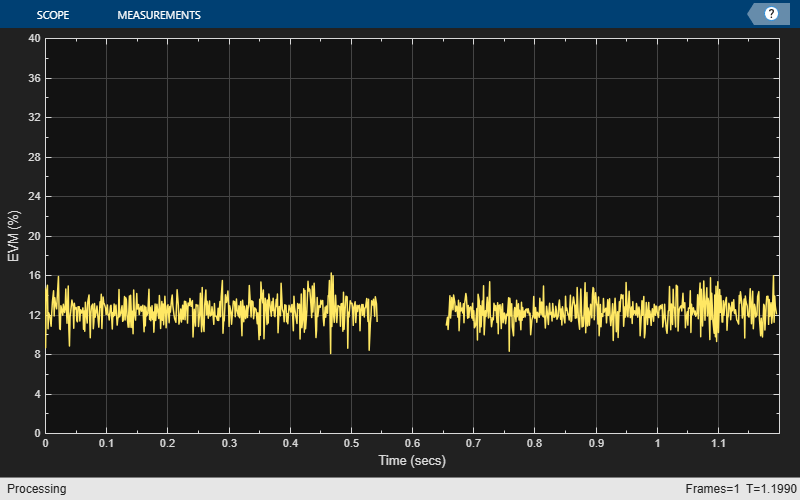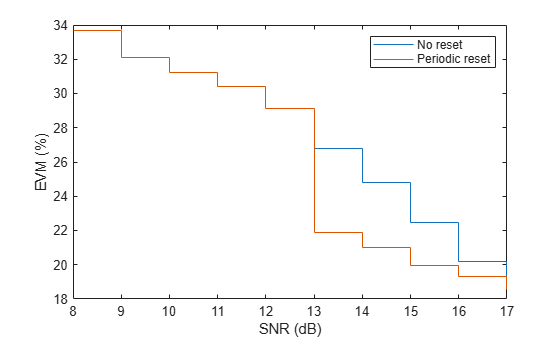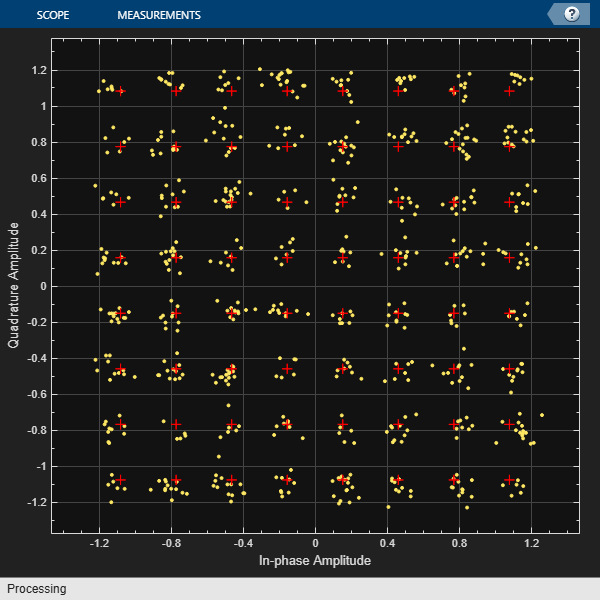# comm.EVM

Measure error vector magnitude (EVM) of received signal

## Description

The `comm.EVM` System object™ measures the root mean squared (RMS) EVM, maximum EVM, and percentile EVM of a received signal.

To measure the EVM of a received signal:

1. Create the `comm.EVM` object and set its properties.

2. Call the object with arguments, as if it were a function.

## Creation

### Syntax

``evm = comm.EVM``
``evm = comm.EVM(Name=Value)``

### Description

example

````evm = comm.EVM` creates an EVM measurement System object.```

example

````evm = comm.EVM(Name=Value)` sets properties using one or more name-value arguments. For example, ```comm.EVM(ReferenceSignalSource="Estimated from reference constellation")``` configures the object to measure the EVM of a received signal relative to a reference constellation.```

## Properties

expand all

Unless otherwise indicated, properties are nontunable, which means you cannot change their values after calling the object. Objects lock when you call them, and the `release` function unlocks them.

If a property is tunable, you can change its value at any time.

Normalization method used in EVM calculation, specified as ```'Average reference signal power'```, `'Average constellation power'`, or `'Peak constellation power'`.

Data Types: `char` | `string`

Average constellation power in Watts, specified as a positive scalar.

#### Dependencies

To enable this property, set the `Normalization` property to ```'Average constellation power'```.

Data Types: `single` | `double` | `int8` | `int16` | `int32` | `int64` | `uint8` | `uint16` | `uint32` | `uint64`

Peak constellation power in Watts, specified as a positive scalar.

#### Dependencies

To enable this property, set the `Normalization` property to ```'Peak constellation power'```.

Data Types: `single` | `double` | `int8` | `int16` | `int32` | `int64` | `uint8` | `uint16` | `uint32` | `uint64`

Reference signal source, specified as `'Input port'` or `'Estimated from reference constellation'`. To provide an explicit reference signal against which to measure received signal, set this property to `'Input port'`. To measure the EVM of the received signal against a reference constellation, set this property to ```'Estimated from reference constellation'```.

Data Types: `char` | `string`

Reference constellation, specified as a vector. The default value corresponds to a quadrature phase-shift keying (QPSK) constellation with unit average power. You can derive constellation points by using modulation functions or objects. For example, to derive the reference constellation for a 16-point quadrature amplitude modulated (16-QAM) signal, use the `qammod` function.

Example: `qammod(0:15,16)`

#### Dependencies

To enable this property, set the `ReferenceSignalSource` property to ```'Estimated from reference constellation'```.

Data Types: `double`
Complex Number Support: Yes

Measurement interval source for RMS and maximum EVM measurements, specified as one of these values.

• `'Input length'` — Measure EVM using only the current samples.

• `'Entire history'` — Measure EVM for all samples.

• `'Custom'` — Measure EVM over an interval you specify and use a sliding window.

• `'Custom with periodic reset'` — Measure EVM over an interval you specify and reset the object after measuring over each interval.

Data Types: `char` | `string`

Measurement interval, specified as a positive integer.

#### Dependencies

To enable this property, set the `MeasurementIntervalSource` property to `'Custom'` or `'Custom with periodic reset'`.

Data Types: `single` | `double` | `int8` | `int16` | `int32` | `int64` | `uint8` | `uint16` | `uint32` | `uint64`

Averaging dimensions over which the object averages the EVM measurements, specified as a vector of integers in the range [1, 3]. For example, to average across the rows, set this property to `2`.

This object supports variable-size inputs of the dimensions across which the averaging takes place. However, the input size for the non-averaged dimensions must remain constant between calls to the object. For example, if the input has size `[1000 3 2]` and you set this property to `[1 3]`, the output size is `[1 3 1]`, and the number of elements in the second dimension must remain fixed at `3`.

Data Types: `single` | `double` | `int8` | `int16` | `int32` | `int64` | `uint8` | `uint16` | `uint32` | `uint64`

Option to return maximum EVM measurements, specified as a logical `1` (`true`) or `0` (`false`).

Data Types: `logical`

Option to return X-percentile EVM measurements, that is, the value below which X% of EVM measurements fall, specified as a logical `1` (`true`) or `0` (`false`). When you set this property to `1` (`true`), X-percentile EVM measurements persist until you reset the object. The object performs these measurements by using all of the input frames since the last reset.

Data Types: `logical`

Value below which X% of EVM measurements fall, specified as a scalar in the range [0, 100].

#### Dependencies

To enable this property, set the `XPercentileEVMOutputPort` property to `true`.

Data Types: `single` | `double` | `int8` | `int16` | `int32` | `int64` | `uint8` | `uint16` | `uint32` | `uint64`

Option to return the number of accumulated symbols that the object uses to measure the X-percentile EVM since the last reset, specified as a logical `1` (`true`) or `0` (`false`).

#### Dependencies

To enable this property, set the `XPercentileEVMOutputPort` property to `true`.

Data Types: `logical`

## Usage

### Syntax

``rmsEVM = evm(refSym,rxSym)``
``[rmsEVM,maxEVM] = evm(refSym,rxSym)``
``[___,xEVM] = evm(refSym,rxSym)``
``[___,numSym] = evm(refSym,rxSym)``
``[___] = evm(rxSym)``

### Description

example

````rmsEVM = evm(refSym,rxSym)` measures the percentage RMS EVM of received signal `rxSym` relative to reference signal `refSym` over the measurement interval specified in the `MeasurementIntervalSource` and `MeasurementInterval` properties.```
````[rmsEVM,maxEVM] = evm(refSym,rxSym)` also measures the maximum percentage EVM over the configured measurement interval.To use this syntax, set the `MaximumEVMOutputPort` property to `true`.```
````[___,xEVM] = evm(refSym,rxSym)` also measures the value below which X% of EVM measurements fall using all input frames since the last reset, regardless of measurement interval configuration. Set the value of X in the `XPercentileValue` property. For example, if you set the `XPercentileValue` to `95`, then 95% of all EVM measurements since the last reset fall below the value of `xEVM`. You can use this syntax with any previous output argument combination.To use this syntax, set the `XPercentileEVMOutputPort` property to `true`.```

example

````[___,numSym] = evm(refSym,rxSym)` also returns the number of symbols used to measure the X-percentile EVM. To use this syntax, set the `XPercentileEVMOutputPort` and `SymbolCountOutputPort` properties to `true`.```

example

````[___] = evm(rxSym)` measures the EVM of the received signal relative to the reference signal specified in the `ReferenceConstellation` property. You can use this syntax with any previous output argument combination.To use this syntax, set the `ReferenceSignalSource` property to ```'Estimated from reference constellation'``` and the `ReferenceConstellation` property to a vector of length equal to that of the `rxSym` input.```

### Input Arguments

expand all

Reference signal, specified as a scalar, vector, matrix, or 3-D array. If you specify this input, the object measures the EVM of the `rxSym` input by using this input as a reference constellation.

The dimensions of this input must match those of the `rxSym` input. The object uses each element of this input as the reference symbol for the corresponding element of the `rxSym` input.

Data Types: `single` | `double` | `int8` | `int16` | `int32` | `int64` | `uint8` | `uint16` | `uint32` | `uint64`
Complex Number Support: Yes

Received signal, specified as a scalar, vector, matrix, or 3-D array.

Data Types: `single` | `double` | `int8` | `int16` | `int32` | `int64` | `uint8` | `uint16` | `uint32` | `uint64`
Complex Number Support: Yes

### Output Arguments

expand all

Percentage RMS EVM of the received signal over the configured measurement interval, returned as a scalar.

Data Types: `double`

Maximum percentage EVM over the configured measurement interval, returned as a scalar.

Data Types: `double`

Value below which X% of EVM measurements fall since the last reset, returned as a scalar in the range [0, 100]. Set the value of X in the `XPercentileValue` property.

Data Types: `double`

Number of symbols that the object uses to measure the `xEVM` output, returned as a positive integer.

Data Types: `double`

## Object Functions

To use an object function, specify the System object as the first input argument. For example, to release system resources of a System object named `obj`, use this syntax:

`release(obj)`

expand all

 `step` Run System object algorithm `release` Release resources and allow changes to System object property values and input characteristics `reset` Reset internal states of System object

## Examples

collapse all

Generate random data symbols and apply 8-PSK modulation.

```d = randi([0 7],2000,1); txSig = pskmod(d,8,pi/8);```

Pass the modulated signal through an additive white Gaussian noise (AWGN) channel.

`rxSig = awgn(txSig,30);`

Create an EVM object. Measure the RMS EVM using the transmitted signal as the reference.

```evm = comm.EVM; rmsEVM1 = evm(txSig,rxSig);```

Release the EVM measurement System object. Configure the object to estimate the EVM of the received signal against a reference constellation.

```release(evm) evm.ReferenceSignalSource = "Estimated from reference constellation"; evm.ReferenceConstellation = pskmod(0:7,8,pi/8);```

Measure the RMS EVM using only the received signal as an input, and then verify that the two EVM results match.

```rmsEVM2 = evm(rxSig); isequal(rmsEVM1,rmsEVM2)```
```ans = logical 1 ```

Create OFDM modulator and demodulator System objects.

```ofdmmod = comm.OFDMModulator(FFTLength=32,NumSymbols=4); ofdmdemod = comm.OFDMDemodulator(FFTLength=32,NumSymbols=4);```

Determine the number of subcarriers and symbols in the OFDM signal.

```ofdmDims = info(ofdmmod); numSC = ofdmDims.DataInputSize(1); numSym = ofdmDims.DataInputSize(2);```

Generate random symbols and apply QPSK modulation.

```msg = randi([0 3],numSC,numSym); rxSym = pskmod(msg,4,pi/4);```

OFDM modulate the QPSK signal. Pass the signal through an AWGN channel. Demodulate the noisy signal.

```txSig = ofdmmod(rxSym); rxSig = awgn(txSig,10,"measured"); refSym = ofdmdemod(rxSig);```

Configure an EVM measurement System object to average the EVM measurement over the subcarriers. Measure the EVM. The four entries correspond to each of the four OFDM symbols.

```evm = comm.EVM(AveragingDimensions=1); rmsEVM = evm(refSym,rxSym)```
```rmsEVM = 1×4 26.4681 25.4270 25.8847 25.6017 ```

Configure the EVM measurement System object to average the EVM measurement over the OFDM symbols. Measure the EVM. The 21 entries correspond to each of the 21 subcarriers.

```evm = comm.EVM(AveragingDimensions=2); rmsEVM = evm(refSym,rxSym); disp(rmsEVM')```
``` 25.8258 27.2699 19.9041 24.3970 27.0605 28.8856 32.7832 17.0630 21.2569 25.5982 23.0833 37.3233 31.1625 25.1941 18.2289 31.0715 26.6774 13.0369 19.0392 38.1465 24.4323 ```

Measure the EVM and average over both the subcarriers and the OFDM symbols.

```evm = comm.EVM(AveragingDimensions=[1 2]); rmsEVM = evm(refSym,rxSym)```
```rmsEVM = 25.8380 ```

Calculate and plot the EVM of an OFDM signal. The signal consists of two packets separated by an interval.

Create System objects to:

• OFDM-modulate a signal.

• Introduce phase noise.

• Plot time-varying signals.

```ofdmmod = comm.OFDMModulator(FFTLength=256,NumSymbols=2); pnoise = comm.PhaseNoise(Level=-60,FrequencyOffset=20,SampleRate=1000); tscope = timescope(YLabel="EVM (%)",YLimits=[0 40], ... SampleRate=1000,TimeSpanSource="Property",TimeSpan=1.2, ... ShowGrid=true);```

Configure an EVM measurement System object to generate a time-varying estimate of the EVM.

```evm = comm.EVM(MaximumEVMOutputPort=false, ... ReferenceSignalSource="Input port", ... AveragingDimensions=2);```

Determine the input data dimensions of the OFDM modulator.

`modDims = info(ofdmmod);`

Create QPSK-modulated random data for the first packet. Apply OFDM modulation.

```data = randi([0 3],modDims.DataInputSize); qpskSig = pskmod(data,4,pi/4); txSig1 = ofdmmod(qpskSig);```

Create a second data packet.

```data = randi([0 3],modDims.DataInputSize); qpskSig = pskmod(data,4,pi/4); txSig2 = ofdmmod(qpskSig);```

Concatenate the two packets and include an interval with no transmitted data.

`txSig = [txSig1; zeros(112,1); txSig2];`

Apply I/Q amplitude and phase imbalance to the transmitted signal.

`rxSigIQimb = iqimbal(txSig,2,5);`

Apply phase noise.

`rxSig = pnoise(rxSigIQimb);`

Measure and plot the EVM of the received signal.

```e = evm(txSig,rxSig); tscope(e)```Configure an EVM object to output maximum EVM, 90th percentile EVM, and symbol count.

```evm = comm.EVM(MaximumEVMOutputPort=true, ... XPercentileEVMOutputPort=true,XPercentileValue=90, ... SymbolCountOutputPort=true);```

Generate random data symbols. Apply 16-QAM modulation. The modulated signal serves as the reference for the subsequent EVM measurements.

```data = randi([0 15],1000,1); refSym = qammod(data,16,UnitAveragePower=true);```

Pass the modulated signal through an AWGN channel.

`rxSym = awgn(refSym,20);`

Measure the EVM of the noisy signal.

`[rmsEVM,maxEVM,pctEVM,numSym] = evm(refSym,rxSym)`
```rmsEVM = 9.8775 ```
```maxEVM = 34.3113 ```
```pctEVM = 15.0200 ```
```numSym = 1000 ```

Measure the EVM of a noisy 8-PSK signal using two types of custom measurement interval and display the results.

Set the number of frames, `M`, and the number of subframes per frame, `K`.

```M = 2; K = 5;```

Set the number of symbols in a subframe. Calculate the corresponding frame length.

```sfLen = 100; frmLen = K*sfLen;```

Create an EVM measurement System object, specifying a custom measurement interval equal to the frame length.

```evm1 = comm.EVM(MeasurementIntervalSource="Custom", ... MeasurementInterval=frmLen);```

Configure the object to measure EVM using an 8-PSK reference constellation.

```evm1.ReferenceSignalSource = "Estimated from reference constellation"; evm1.ReferenceConstellation = pskmod(0:7,8,pi/8);```

Create an EVM measurement System object, specifying a 500-symbol measurement interval with a periodic reset. Configure the object to measure EVM using an 8-PSK reference constellation.

```evm2 = comm.EVM(... MeasurementIntervalSource="Custom with periodic reset", ... MeasurementInterval=frmLen); evm2.ReferenceSignalSource = ... "Estimated from reference constellation"; evm2.ReferenceConstellation = pskmod(0:7,8,pi/8);```

Initialize the EVM and signal-to-noise ratio (SNR) arrays.

```rmsEVM1 = zeros(K,M); rmsEVM2 = zeros(K,M); snrdB = zeros(K,M);```

Measure the EVM for a noisy 8-PSK signal using both objects. The SNR increases by 1 dB from subframe to subframe. The `evm1` object uses the 500 most recent symbols to compute the estimate. In this case, the object uses a sliding window so that it processes an entire frame of data. The `evm2` object clears the symbols each time it begins processing a new frame.

```for m = 1:M for k = 1:K data = randi([0 7],sfLen,1); txSig = pskmod(data,8,pi/8); snrdB(k,m) = k + (m-1)*K + 7; rxSig = awgn(txSig,snrdB(k,m)); rmsEVM1(k,m) = evm1(rxSig); rmsEVM2(k,m) = evm2(rxSig); end end```

Display the EVM measured using the two approaches. The windowing used in the first case provides an averaging across the subframes. In the second case, the EVM object resets after the first frame so that the calculated EVM values more accurately reflect the current SNR.

```stairs(snrdB(:),[rmsEVM1(:) rmsEVM2(:)]) xlabel('SNR (dB)') ylabel('EVM (%)') legend('No reset','Periodic reset')```Generate filtered QAM data and pass it through an AWGN channel. Compute the symbol error rate, and estimate the EVM of the received signal.

Create channel and filter System objects.

```M = 16; refConst = qammod(0:M-1,M); channel = comm.AWGNChannel( ... NoiseMethod="Signal to noise ratio (SNR)", ... SNR=15,SignalPower=10); txfilter = comm.RaisedCosineTransmitFilter(OutputSamplesPerSymbol=4); rxfilter = comm.RaisedCosineReceiveFilter(InputSamplesPerSymbol=4, ... DecimationFactor=4);```

Create an EVM measurement System object to calculate RMS and maximum EVM.

```evm = comm.EVM(MaximumEVMOutputPort=true, ... ReferenceSignalSource="Estimated from reference constellation", ... ReferenceConstellation=refConst);```

Create an error rate measurement System object and account for the signal delay through the transmit and receive filters. For a filter, the group delay is equal to half of the `FilterSpanInSymbols` property.

```rxd = (txfilter.FilterSpanInSymbols + rxfilter.FilterSpanInSymbols)/2; errorRate = comm.ErrorRate(ReceiveDelay=rxd);```

Perform these channel operations:

• Generate random data symbols.

• Apply 16-QAM.

• Filter the modulated data through a raised cosine transmit filter.

• Pass the transmitted signal through an AWGN channel.

• Demodulate the filtered data.

```txData = randi([0 15],1000,1); modData = qammod(txData,M); txSig = txfilter(modData); rxSig = channel(txSig); filtSig = rxfilter(rxSig); rxData = qamdemod(filtSig,M);```

Calculate the error statistics and display the symbol error rate.

```errStats = errorRate(txData,rxData); symErrRate = errStats(1)```
```symErrRate = 0.0222 ```

Measure and display the received RMS EVM and maximum EVM values. Take the filter delay into account by deleting the first `rxd+1` symbols. Because the received signal contains symbol errors, the EVM might not be totally accurate.

`[rmsEVM,maxEVM] = evm(filtSig(rxd+1:end))`
```rmsEVM = 17.2966 ```
```maxEVM = 40.1595 ```

Measure the RMS and maximum EVM of a distorted OFDM waveform and visualize the received symbols by using a constellation diagram.

Generate an OFDM waveform with 64-QAM for random data.

```M = 64; nfft = 64; nSym = 10; cpLen = 10; data = randi([0 (M - 1)]',nfft,nSym); txSym = qammod(data,M,UnitAveragePower=1); txWaveform = ofdmmod(txSym,nfft,cpLen);```

Apply nonlinear distortion to the signal by creating a memoryless nonlinearity System object.

```gain = 2; nonlinearity = comm.MemorylessNonlinearity(Method="Rapp model", ... LinearGain=gain); rxWaveform = nonlinearity(txWaveform);```

Recover the distorted symbols by performing OFDM demodulation.

`rxSym = ofdmdemod(rxWaveform,nfft,cpLen);`

Measure the RMS and maximum EVM per OFDM symbol of the received signal.

```refSym = qammod((0:(M - 1))',M,UnitAveragePower=1); evm = comm.EVM(MaximumEVMOutputPort=1, ... ReferenceSignalSource="Estimated from reference constellation", ... ReferenceConstellation=refSym, ... Normalization="Average constellation power"); [rmsEVM,maxEVM] = evm(rxSym); disp(rmsEVM')```
``` 8.0933 7.4962 7.7542 7.7335 7.5719 7.9262 7.7042 8.6034 8.0817 7.6852 ```
`disp(maxEVM')`
``` 18.8246 15.6412 15.9905 13.0713 14.0164 15.5771 17.0201 19.4699 18.2106 16.7549 ```

Visualize the received symbols on a constellation diagram.

```constellation = comm.ConstellationDiagram( ... Name="Constellation Diagram of Received Symbols", ... ReferenceConstellation=refSym(:)); constellation(rxSym(:))```## Algorithms

The implementation supports three normalization methods. You can normalize measurements according to the average power of the reference signal, average constellation power, or peak constellation power. Different industry standards follow one of these normalization methods.

The algorithm calculates the RMS EVM value differently for each normalization method.

EVM Normalization MethodAlgorithm
Reference signal

`$EV{M}_{\text{RMS}}=\sqrt{\frac{\frac{1}{N}\sum _{k=1}^{N}\left({e}_{k}\right)}{\frac{1}{N}\sum _{k=1}^{N}\left({I}_{k}^{2}+{Q}_{k}^{2}\right)}}×100$`

Average power

`$EV{M}_{\text{RMS}}\left(\text{%}\right)=100\sqrt{\frac{\frac{1}{N}\sum _{k=1}^{N}\left({e}_{k}\right)}{{P}_{\text{avg}}}}$`

Peak power

`$EV{M}_{\text{RMS}}\left(\text{%}\right)=100\sqrt{\frac{\frac{1}{N}\sum _{k=1}^{N}\left({e}_{k}\right)}{{P}_{\text{max}}}}$`

In these equations:

• ek = ${e}_{k}={\left({I}_{k}-{\stackrel{˜}{I}}_{k}\right)}^{2}+{\left({Q}_{k}-{\stackrel{˜}{Q}}_{k}\right)}^{2}$

• Ik is the in-phase measurement of the kth symbol in the burst.

• Qk is the quadrature phase measurement of the kth symbol in the burst.

• N is the input vector length.

• Pavg is the average constellation power.

• Pmax is the peak constellation power.

• Ik and Qk represent ideal (reference) values. ${\stackrel{˜}{I}}_{k}$ and ${\stackrel{˜}{Q}}_{k}$ represent measured (received) symbols.

The maximum EVM is the maximum EVM value in a frame or $EV{M}_{\text{max}}=\underset{k\in \left[1,...,N\right]}{\text{max}}\left\{EV{M}_{k}\right\}\text{\hspace{0.17em}}\text{,}$ where k is the kth symbol in a burst of length N.

The definition for EVMk depends on which normalization method you select for computing measurements. The implementation supports these algorithms.

EVM Normalization MethodAlgorithm
Reference signal

`$EV{M}_{k}=\sqrt{\frac{{e}_{k}}{\frac{1}{N}\sum _{k=1}^{N}\left({I}_{k}^{2}+{Q}_{k}^{2}\right)}}×100$`

Average power

`$EV{M}_{k}=100\sqrt{\frac{{e}_{k}}{{P}_{\text{avg}}}}$`

Peak power

`$EV{M}_{k}=100\sqrt{\frac{{e}_{k}}{{P}_{\text{max}}}}$`

The implementation computes the X-percentile EVM by creating a histogram of the incoming EVMk values. This output provides the EVM value below which X% of the EVM values fall.

## Version History

Introduced in R2012a